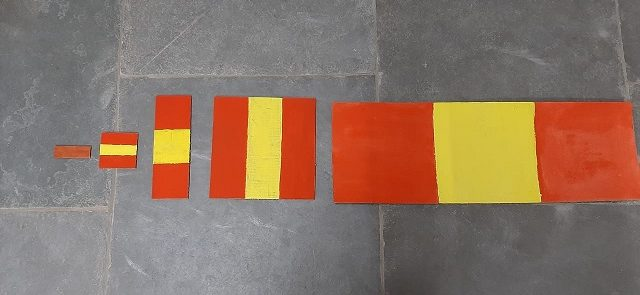# Powers of Three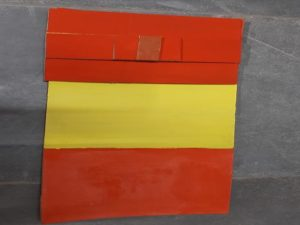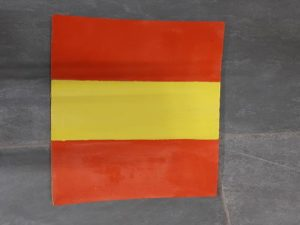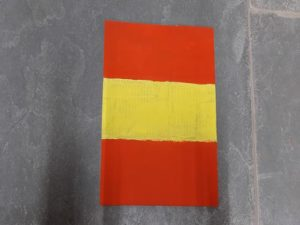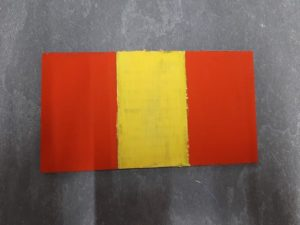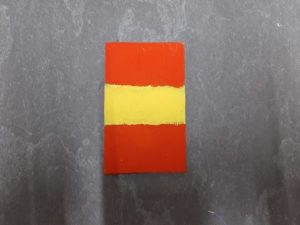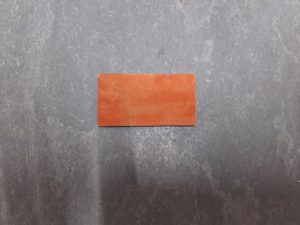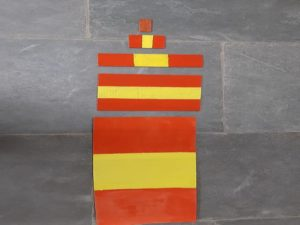-Pratap & Logeshwari

Children from 8th  in Udavi were learning about the powers and exponents.  In order to demonstrate powers of three. I was thinking about how to make the cubes and I had a question of with what materials do I need to make.Finally, I thought of making the cube using Dienes blocks. I took all the 10 cm rods and started. making making the cubes. I wanted to show  31  to  35.

I took a 10 cm rod and cut them into 3 pieces of  3 cm. I stuck them together to get 3^2. when I add three of the 3^2 I get 3^3.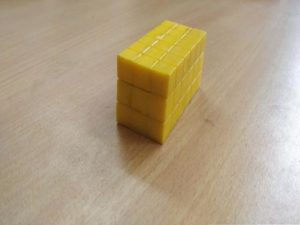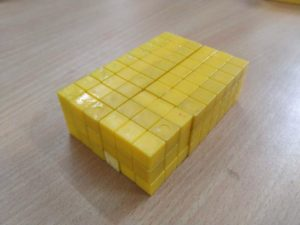Here are some simple rules to use with exponents.

1. a1 = a
Any number raised to the power of one equals the number itself.
2. For any number a, except 0, a0 = 1
Any number raised to the power of zero, except zero, equals one.
3. For any numbers a, b, and c,
ab x ac = ab+c

This multiplication rule tells us that we can simply add the exponents when multiplying two powers with the same base.

The above is a visualizing 3 power n in three dimensions. We did the same for two-dimension also.

We had a session with Ravi Alungati with all the teachers who work on Mathematics. Everyone chose their own topic that they are working on. Pratap and I chose powers since the eighth graders are working on it. We built these two dimensions of representation using the cardboard. We marked the cardboard cut them and painted them in alternate colors.